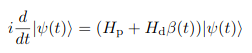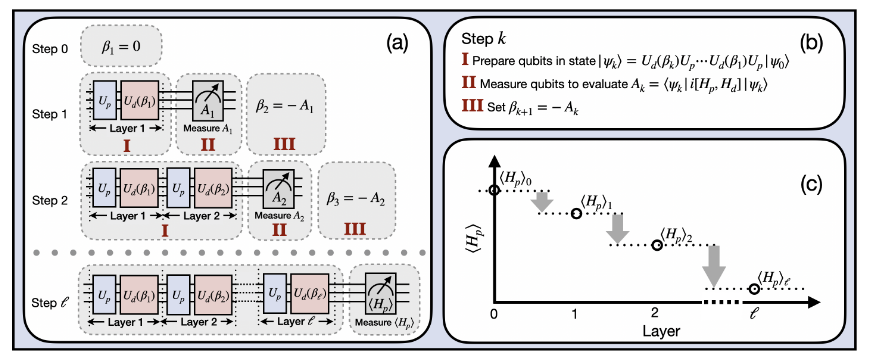# Feedback-based ALgorithm for Quantum OptimizatioN - FALQON#

Quantum Approximate Optimisation Algorithm (QAOA) is considered as one of the most important algorithms for optimisation in Quantum Computers, see arXiv:1411.4028 by Farhi, Goldstone and Gutmann for more information.

In this QAOA algorithm, the aim is to have a problem Hamiltonian H_P and a mixer Hamiltonian H_B. Then, starting with the ground state of H_B, the goal is to repeatedly apply e^(i H_P c) and e^(i H_B b), where c and b are tunable parameters. The values of such parameters are to be found via classical optimization

In the FALQON algorithm, arXiv:2103.08619 by Magann, Rudinger, Grace and Sarovan, they propose a similar although conceptually different algorithm. The proposal consists in evolving the initial state using the Schrödinger equationThis equation satisfies that the expectation value of H_P is monotonically decreasing. This feature is used to create a Hamiltonian evolution with 1 layer using e^(i H_P c) and e^(i H_B b). In the first layer, b=0, and c is a parameter to be defined. Then, the quantity A = i[H_P, H_B] is measured. Its expectation value is then taken to be the parameter b for the next layer. As more layers are added, the approximation to the ground state of the problem Hamiltonian H_P is more and more accurate.## Running the code#

This example contains just one file

• `main.py` is the file where the algorithm is run. The main class `FALQON` is now introduced in `QIBO`

The `FALQON` class behaves similarly to the `QAOA` one. It admits the following parameters:

• `hamiltonian`: problem Hamiltonian whose ground state is sought.

• `mixer`: mixer Hamiltonian. If `None`, `qibo.hamiltonians.X` is used.

• `solver`: solver used to apply the exponential operators. Default solver is ‘exp’.

• `callbacks`: List of callbacks to calculate during evolution.

• `accelerators`: Dictionary of devices to use for distributed execution. See `qibo.core.distcircuit.DistributedCircuit` for more details. This option is available only when `hamiltonian` is a `qibo.abstractions.hamiltonians.TrotterHamiltonian`.

• `memory_device`: Name of device where the full state will be saved. Relevant only for distributed execution (when `accelerators` is given).

When performing the execution of the problem, the following variables are to be set:

• `delta_t`: initial guess for the time step. A too large delta_t will make the algorithm fail.

• `max_layers`: maximum number of layers allowed for the FALQON.

• `initial_state`: initial state vector of the FALQON.

• `tol`: Tolerance of energy change. If not specified, no check is done.

• `callback`: Called after each iteration for scipy optimizers.

• `options`: a dictionary with options for the different optimizers.

• `compile`: whether the TensorFlow graph should be compiled.

• `processes`: number of processes when using the paralle BFGS method.

The attached example provides an easy implementation of the FALQON method for a Heisenberg XXZ model.